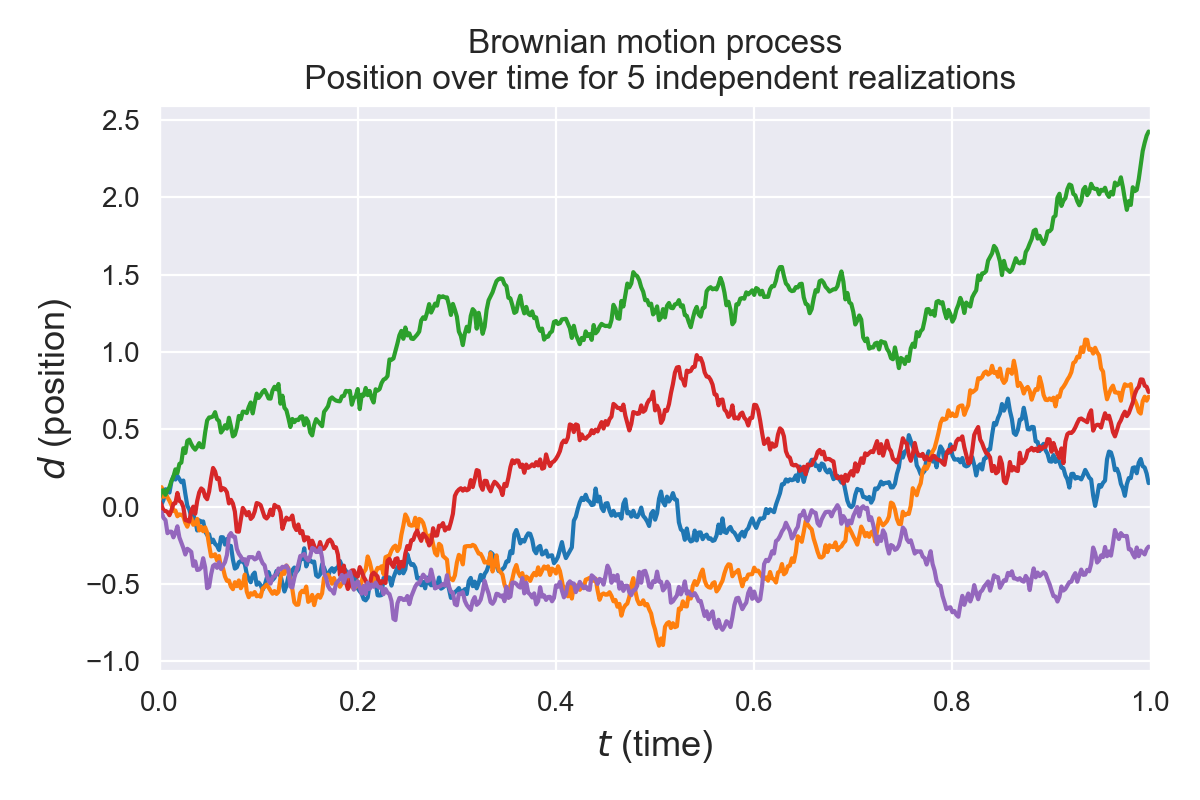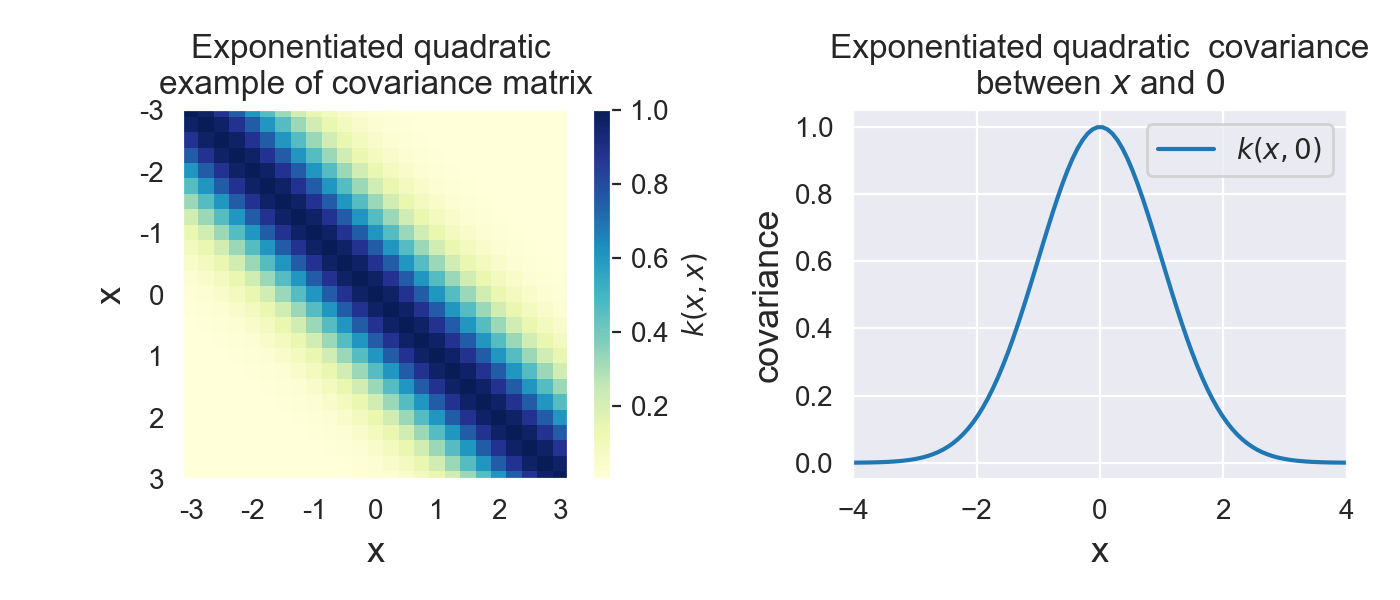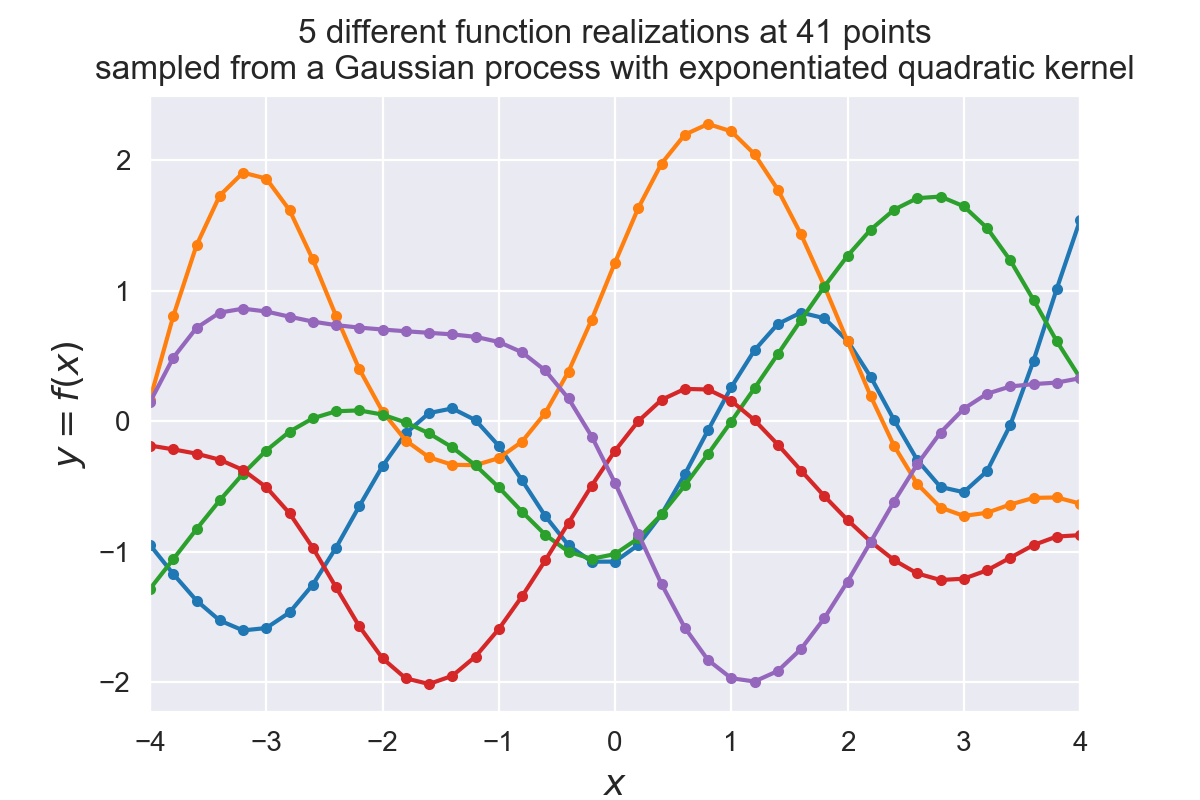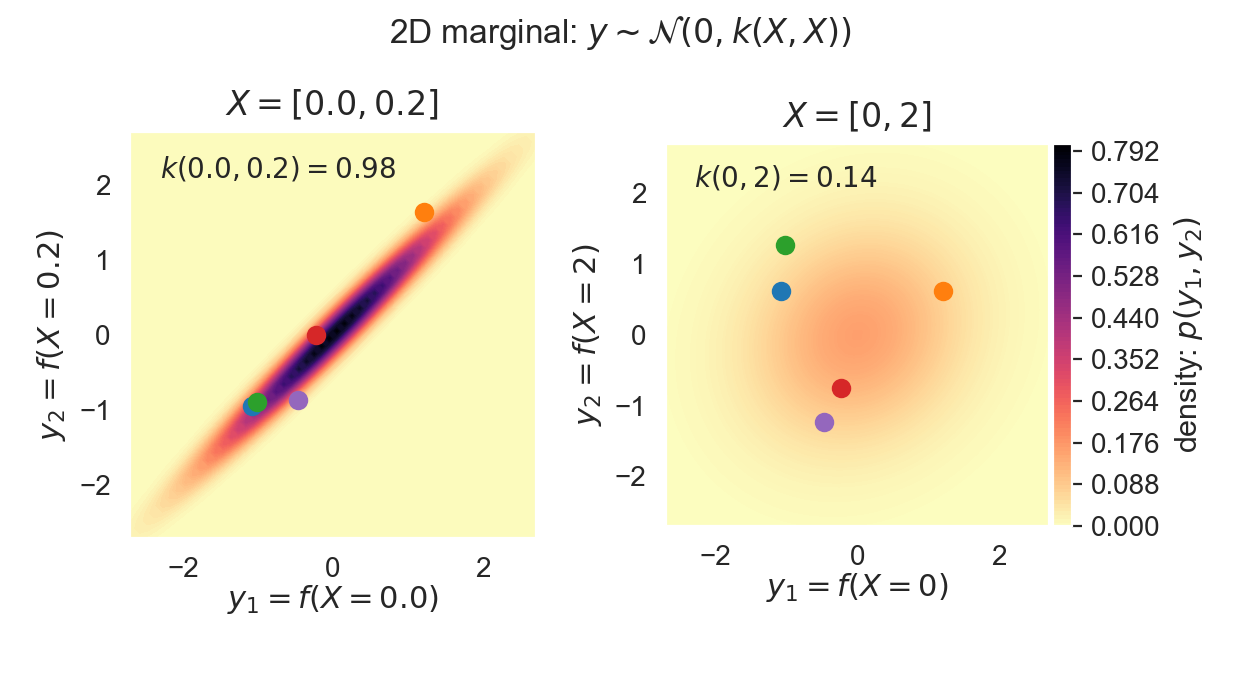# Understanding Gaussian processes

This post explores some of the concepts behind Gaussian processes such as stochastic processes and the kernel function. We will build up deeper understanding on how to implement Gaussian process regression from scratch on a toy example.

This post is followed by a second post demonstrating how to fit a Gaussian process kernel with TensorFlow probability . This post is part of series on Gaussian processes:

In what follows we assume familiarity with basic probability and linear algebra especially in the context of multivariate Gaussian distributions. Have a look at this post if you need a refresher on the Gaussian distribution.

In :

What are Gaussian processes? The name implies that its a stochastic process of random variables with a Gaussian distribution. This might not mean much at this moment so lets dig a bit deeper in its meaning.

## Stochastic process ¶

Stochastic processes typically describe systems randomly changing over time. The processes are stochastic due to the uncertainty in the system. Even if the starting point is known, there are several directions in which the processes can evolve.

An example of a stochastic process that you might have come across is the model of Brownian motion (also known as Wiener process ). Brownian motion is the random motion of particles suspended in a fluid. It can be seen as a continuous random walk where a particle moves around in the fluid due to other particles randomly bumping into it. We can simulate this process over time $t$ in 1 dimension $d$ by starting out at position 0 and move the particle over a certain amount of time $\Delta t$ with a random distance $\Delta d$ from the previous position.The random distance is sampled from a normal distribution with mean $0$ and variance $\Delta t$. Sampling $\Delta d$ from this normal distribution is noted as $\Delta d \sim \mathcal{N}(0, \Delta t)$. The position $d(t)$ at time $t$ evolves as $d(t + \Delta t) = d(t) + \Delta d$.

We simulate 5 different paths of brownian motion in the following figure, each path is illustrated with a different color.

In :### Stochastic processes as distributions over functions ¶

Notice in the figure above that the stochastic process can lead to different paths, also known as realizations of the process. Each realization defines a position $d$ for every possible timestep $t$. Every realization thus corresponds to a function $f(t) = d$.

This means that a stochastic process can be interpreted as a random distribution over functions. We can sample a realization of a function from a stochastic process. However each realized function can be different due to the randomness of the stochastic process.

Like the model of Brownian motion, Gaussian processes are stochastic processes. In fact, the Brownian motion process can be reformulated as a Gaussian process ⁽³⁾ .

## Gaussian processes ¶

Gaussian processes are distributions over functions $f(x)$ of which the distribution is defined by a mean function $m(x)$ and positive definite covariance function $k(x,x')$, with $x$ the function values and $(x,x')$ all possible pairs in the input domain :

$$f(x) \sim \mathcal{GP}(m(x),k(x,x'))$$

where for any finite subset $X =\{\mathbf{x}_1 \ldots \mathbf{x}_n \}$ of the domain of $x$, the marginal distribution is a multivariate Gaussian distribution:

$$f(X) \sim \mathcal{N}(m(X), k(X, X))$$

with mean vector $\mathbf{\mu} = m(X)$ and covariance matrix $\Sigma = k(X, X)$.

While the multivariate Gaussian caputures a finte number of jointly distributed Gaussians, the Gaussian process doesn't have this limitation. Its mean and covariance are defined by a function ). Each input to this function is a variable correlated with the other variables in the input domain, as defined by the covariance function. Since functions can have an infinite input domain, the Gaussian process can be interpreted as an infinite dimensional Gaussian random variable.

### Covariance function as prior ¶

To sample functions from the Gaussian process we need to define the mean and covariance functions. The covariance function $k(x_a, x_b)$ models the joint variability of the Gaussian process random variables. It returns the modelled covariance between each pair in $x_a$ and $x_b$.

The specification of this covariance function, also known as the kernel function, implies a distribution over functions $f(x)$. By choosing a specific kernel function $k$ it is possible to set prior information on this distribution. This kernel function needs to be positive-definite in order to be a valid covariance function.

In this post we will model the covariance with the exponentiated quadratic covariance function (also known as the RBF kernel):

$$k(x_a, x_b) = \exp{ \left( -\frac{1}{2\sigma^2} \lVert x_a - x_b \rVert^2 \right)}$$

Other kernel function can be defined resulting in different priors on the Gaussian process distribution. Examples of different kernels are given in a follow up post .

In :
# Define the exponentiated quadratic
# L2 distance (Squared Euclidian)
sq_norm = -0.5 * scipy.spatial.distance.cdist(xa, xb, 'sqeuclidean')
return np.exp(sq_norm)


An example covariance matrix from the exponentiated quadratic covariance function is plotted in the figure below on the left. The covariance vs input zero is plotted on the right. Note that the exponentiated quadratic covariance decreases exponentially the further away the function values $x$ are from eachother.

In :### Sampling from prior ¶

In practice we can't just sample a full function evaluation $f$ from a Gaussian process distribution since that would mean evaluating $m(x)$ and $k(x,x')$ at an infinite number of points since $x$ can have an infinite domain . We can however sample function evaluations $\mathbf{y}$ of a function $f$ drawn from a Gaussian process at a finite, but arbitrary, set of points $X$: $\mathbf{y} = f(X)$.

A finite dimensional subset of the Gaussian process distribution results in a marginal distribution that is a Gaussian distribution $\mathbf{y} \sim \mathcal{N}(\mathbf{\mu}, \Sigma)$ with mean vector $\mathbf{\mu} = m(X)$, covariance matrix $\Sigma = k(X, X)$.

In the figure below we will sample 5 different function realisations from a Gaussian process with exponentiated quadratic prior ⁽¹⁾ without any observed data. We do this by drawing correlated samples from a 41-dimensional Gaussian $\mathcal{N}(0, k(X, X))$ with $X = [X_1, \ldots, X_{41}]$.

In :
# Sample from the Gaussian process distribution
nb_of_samples = 41  # Number of points in each function
number_of_functions = 5  # Number of functions to sample
# Independent variable samples
X = np.expand_dims(np.linspace(-4, 4, nb_of_samples), 1)
Σ = exponentiated_quadratic(X, X)  # Kernel of data points

# Draw samples from the prior at our data points.
# Assume a mean of 0 for simplicity
ys = np.random.multivariate_normal(
mean=np.zeros(nb_of_samples), cov=Σ,
size=number_of_functions)

In :Another way to visualise this is to take only 2 dimensions of this 41-dimensional Gaussian and plot some of it's 2D marginal distibutions.

The next figure on the left visualizes the 2D distribution for $X = [0, 0.2]$ where the covariance $k(0, 0.2) = 0.98$. The figure on the right visualizes the 2D distribution for $X = [0, 2]$ where the covariance $k(0, 2) = 0.14$.

For each of the 2D Gaussian marginals the corresponding samples from the function realisations above have plotted as colored dots on the figure.

Observe that points close together in the input domain of $x$ are strongly correlated ($y_1$ is close to $y_2$), while points further away from eachother are almost independent. This is because these marginals come from a Gaussian process with as prior the exponentiated quadratic covariance which adds prior information that points close to eachother in the input space $X$ must be close to eachother in the output space $y$.

In :## Gaussian processes for regression ¶

Since Gaussian processes model distributions over functions we can use them to build regression models. We can treat the Gaussian process as a prior defined by the kernel function and create a posterior distribution given some data. This posterior distribution can then be used to predict the expected value and probability of the output variable $\mathbf{y}$ given input variables $X$.

### Predictions from posterior ¶

We want to make predictions $\mathbf{y}_2 = f(X_2)$ for $n_2$ new samples, and we want to make these predictions based on our Gaussian process prior and $n_1$ previously observed data points $(X_1,\mathbf{y}_1)$. This can be done with the help of the posterior distribution $p(\mathbf{y}_2 \mid \mathbf{y}_1,X_1,X_2)$. Keep in mind that $\mathbf{y}_1$ and $\mathbf{y}_2$ are jointly Gaussian since they both should come from the same function. Since they are jointly Gaussian and we have a finite number of samples we can write:

$$\left[\begin{array}{c} \mathbf{y}_{1} \\ \mathbf{y}_{2} \end{array}\right] \sim \mathcal{N} \left( \left[\begin{array}{c} \mu_{1} \\ \mu_{2} \end{array}\right], \left[ \begin{array}{cc} \Sigma_{11} & \Sigma_{12} \\ \Sigma_{21} & \Sigma_{22} \end{array} \right] \right)$$

Where: $$\begin{split} \mu_{1} & = m(X_1) \quad (n_1 \times 1) \\ \mu_{2} & = m(X_2) \quad (n_2 \times 1) \\ \Sigma_{11} & = k(X_1,X_1) \quad (n_1 \times n_1) \\ \Sigma_{22} & = k(X_2,X_2) \quad (n_2 \times n_2) \\ \Sigma_{12} & = k(X_1,X_2) = k_{21}^\top \quad (n_1 \times n_2) \end{split}$$

Note that $\Sigma_{11}$ is independent of $\Sigma_{22}$ and vice versa.

We can then get the conditional distribution :

$$\begin{split} p(\mathbf{y}_2 \mid \mathbf{y}_1, X_1, X_2) & = \mathcal{N}(\mu_{2|1}, \Sigma_{2|1}) \\ \mu_{2|1} & = \mu_2 + \Sigma_{21} \Sigma_{11}^{-1} (\mathbf{y}_1 - \mu_1) \\ & = \Sigma_{21} \Sigma_{11}^{-1} \mathbf{y}_1 \quad (\text{if assume mean prior } \mu = 0 ) \\ \Sigma_{2|1} & = \Sigma_{22} - \Sigma_{21} \Sigma_{11}^{-1}\Sigma_{12} \end{split}$$

We can write these as follows (Note here that $\Sigma_{11} = \Sigma_{11}^{\top}$ since it's symmetric .):

$$\begin{array}{cc} \begin{split} \mu_{2|1} & = \Sigma_{21} \Sigma_{11}^{-1} \mathbf{y}_1 \\ & = (\Sigma_{11}^{-1} \Sigma_{12})^{\top} \mathbf{y}_1 \\ \end{split} & \qquad \begin{split} \Sigma_{2|1} & = \Sigma_{22} - \Sigma_{21} \Sigma_{11}^{-1} \Sigma_{12} \\ & = \Sigma_{22} - (\Sigma_{11}^{-1} \Sigma_{12})^{\top} \Sigma_{12} \\ \end{split} \end{array}$$

It is then possible to predict $\mathbf{y}_2$ corresponding to the input samples $X_2$ by using the mean $\mu_{2|1}$ of the resulting distribution as a prediction. Notice that the mean of the posterior predictions $\mu_{2|1}$ of a Gaussian process are weighted averages of the observed variables $\mathbf{y}_1$, where the weighting is based on the coveriance function $k$. The variance $\sigma_2^2$ of these predictions is then the diagonal of the covariance matrix $\Sigma_{2|1}$.

The Gaussian process posterior is implemented in the  GP  method below. We can compute the $\Sigma_{11}^{-1} \Sigma_{12}$ term with the help of Scipy's  solve  function ⁽²⁾ .

In :
# Gaussian process posterior
def GP(X1, y1, X2, kernel_func):
"""
Calculate the posterior mean and covariance matrix for y2
based on the corresponding input X2, the observations (y1, X1),
and the prior kernel function.
"""
# Kernel of the observations
Σ11 = kernel_func(X1, X1)
# Kernel of observations vs to-predict
Σ12 = kernel_func(X1, X2)
# Solve
solved = scipy.linalg.solve(Σ11, Σ12, assume_a='pos').T
# Compute posterior mean
μ2 = solved @ y1
# Compute the posterior covariance
Σ22 = kernel_func(X2, X2)
Σ2 = Σ22 - (solved @ Σ12)
return μ2, Σ2  # mean, covariance


The code below calculates the posterior distribution based on 8 observations from a sine function. The results are plotted below. The top figure shows the distribution where the red line is the posterior mean, the grey area is the 95% prediction interval, the black dots are the observations $(X_1,\mathbf{y}_1)$. The prediction interval is computed from the standard deviation $\sigma_{2|1}$, which is the square root of the diagonal of the covariance matrix. The bottom figure shows 5 realizations (sampled functions) from this distribution.

Note that the distrubtion is quite confident of the points predicted around the observations \$(X_1,\math